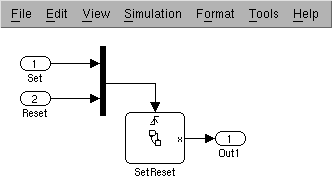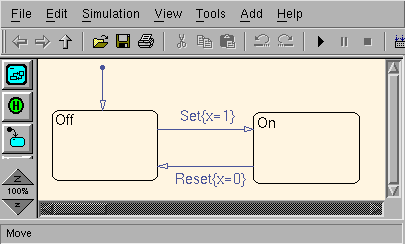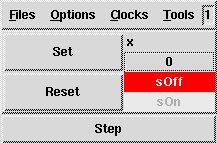## 2  The sf2lus translator

### 2.1  A simple worked exampleFigure 1: A simple Simulink/Stateflow chart

Consider the very simple Stateflow chart shown in Figure 12.

Assume that the Matlab model file is SetReset_r13.mdl. Running the translator in isolation on this model extracts the Stateflow component and generates a Lustre node for each top-level Stateflow chart in the model.
```% sf2lus SetReset_r13.mdl -o SetReset_r13.lus
```
The Lustre nodes are called `sf_<CID>` where `<CID>` is the id field in the Stateflow chart entry. This node looks as follows, noting that the first Stateflow chart in the model almost always has number 2:
```-- graph id=11 name=22,GCTOP
node sf_2(Set, Reset: event) returns(x: int);
var ini, lv5, lv5_1, lv6, lv6_1, lv7, lv7_1, ok22, ok22_1, ok22_2, ok22_3,
s3, s3_1, s3_2, s3t, s4, s4_1, s4_2, s4t, trm: bool; x_1, x_2: int;
let
...
```
This is valid Lustre code and can be compiled with Lustre:
```lustre SetReset_r13.lus sf_2 -o SetReset_r13.oc -0
poc -loop -o SetReset_r13.c SetReset_r13.oc
gcc -c SetReset_r13.c -o SetReset_r13.o
gcc -c SetReset_r13_loop.c -o SetReset_r13_loop.o
gcc SetReset_r13.o SetReset_r13_loop.o -o SetReset_r13
```
The resulting program can be run:
```% SetReset_r13
##### STEP 1 ##################
Set (true=1/false=0) ? 0
Reset (true=1/false=0) ? 0
x = 0
##### STEP 2 ##################
Set (true=1/false=0) ? 1
Reset (true=1/false=0) ? 0
x = 1
##### STEP 3 ##################
Set (true=1/false=0) ? 0
Reset (true=1/false=0) ? 1
x = 0
```
Alternatively, we can simulate the code with Luciole:
```% luciole SetReset_r13.lus sf_2
```
resulting in something like the following:### 2.2  Debugging Stateflow with sf2lus

While this is the required behaviour from the Lustre code it does not say much about the internal state of the running Lustre program. Neither are the generated variables particularly meaningful since states are represented simply by their `id` numbers.

#### 2.2.1  Viewing the internal state

If we wish to use sf2lus as a means of debugging the Stateflow component of a Simulink application then it might be useful both to give sensible names to the variables and to export some of the internal state of the chart, for example the state variables. We can do this as follows:
```% sf2lus -names -states_visible SetReset_r13.mdl -o SetReset_r13.lus
```
resulting in the following Luciole display:Now we can see which states are active. State names are based on the node names in Stateflow preceded by `s` for a terminal state and `sg` for a state containing substates when there is hierarchy or parallel states. Note that these are abbreviated names so, for example, ``state'' is shortened to ``s'' and ``subgraph'' to ``sg''. Use the `-long_names` option to generate the full names which results in voluminous output even for small charts. Note also that with `-long_names` the chart names become `stateflow_<CID>`.

#### 2.2.2  Even more detailed output

In fact, we can go further using the `-temps_visible` or `-locals_visible` options which attempt to dump the entire internal state of the working chart3. For example:For this simple chart the output is small but the output can become prohibitively large for even modestly-sized charts. The most useful elements of this display are the transition validity flags (for example `sf_2_lv5`) which indicate which transition was traversed on the previous step.

#### 2.2.3  Setting multiple boolean inputs with Luciole

One problem with Luciole is that it is not possible to set more than one boolean input simultaneously so sf2lus provides an option `-input_bools_ints` which transforms input booleans into integers. Note that this does not work for input events but Stateflow processes one event at a time so this restriction is not relevant unless, for example, one is model-checking the code. In any case, events can be turned into integers using the `-sends` option discussed in Section 3: